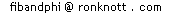# A General pattern for arctan(1/n) = arctan(1/(n+1)) + arctan(1/Z)

Leroy Quet emailed me (Nov 1998) with the correct general pattern:
 arctan ( 1 ) = arctan ( 1 ) + arctan ( 1 )nn+1n(n+1)+1
and also a proof which I have used as the basis of the proof here to make it simpler.

The proof needs another formula about tangents of angles that we have not covered on the Pi and the Fibonacci Numbers page:

 tan(a – b) = tan(a) – tan(b)1 + tan(a)tan(b)
The formula we are trying to prove contains arctans so if we let tan(a) be A and tan(b) be B then we have
a = arctan(A) and b = arctan(B)

and our tan(a-b) formula becomes:
 tan(a – b) = A – B1 + AB
So we can rewrite this equation involving tan in terms of arctan as follows:
 a – b = arctan ( A – B )1 + AB
We can now remove all the angles (small letters) in favour of their tangents (large letters) as so have everything in terms of arctan:
 arctan(A) – arctan(B) = arctan ( A – B )1 + AB
 arctan(A) = arctan(B) + arctan ( A – B )1 + AB
Now we take some special cases which are the ones we are interested in from the original question: let A=1/n and B = 1/(n+1).
Using algebra, you can easily check that the value in the third arctan (A+B)/(1-AB) becomes 1/(n(n+1)+1). So we have our final formula:
 arctan ( 1 ) = arctan ( 1 ) + arctan ( 1 )nn+1n(n+1)+1
which is just what we were after!
We can put this in words as "The final arctan is just 1 more than the product of the other two (whose denominators differ by one)."

Now we need a proof of the formula we began with for tan(a-c). One question remains... How do we prove the formula we started from:

 tan(a – b) = tan(a) – tan(b)1 + tan(a)tan(b)

Back to the original problem on Pi and the Fibonacci Numbers page.
© 1998 - 2014 Dr Ron Knott